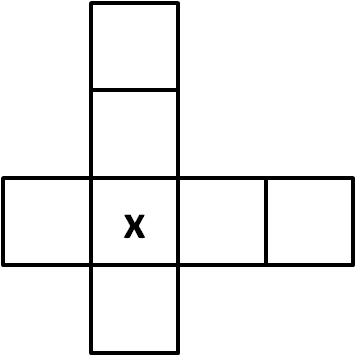#### You may also like### Consecutive Numbers

An investigation involving adding and subtracting sets of consecutive numbers. Lots to find out, lots to explore.### Calendar Capers

Choose any three by three square of dates on a calendar page...### Days and Dates

Investigate how you can work out what day of the week your birthday will be on next year, and the year after...

# Crossover

##### Age 11 to 14 ShortChallenge LevelThe numbers $2$, $3$, $4$, $5$, $6$, $7$ and $8$ are placed in the squares in this diagram.

The four numbers in the horizontal row add up to $21$, and the four numbers in the vertical column also add up to $21$.

Which number should replace $x$?

If you liked this problem, here is an NRICH task that challenges you to use similar mathematical ideas.

This problem is taken from the UKMT Mathematical Challenges.
You can find more short problems, arranged by curriculum topic, in our short problems collection.